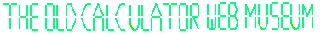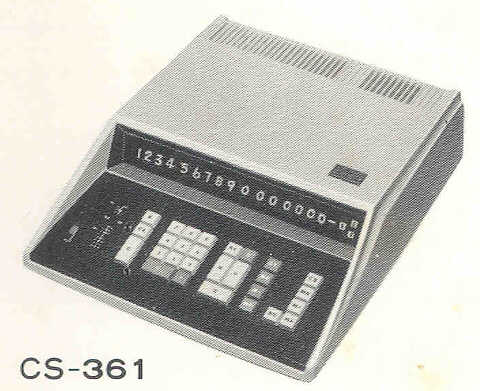+Home Museum Wanted Advertising Articles EMailSharp Compet 361-Series Desktop CalculatorsSharp made a number of different versions of the Compet 361, all of which look the same, but have different functions. All of the models in the 361 series provide 16 digits of capacity; addition, subtraction, multiplication, and division; constant multiplication and divison; two accumulator-style memory registers; leading zero suppression; combination of small-scale MOS integrated circuitry and transistorized logic; Nixie tube display; and magnetic core memory for register storage. The initial models in the series were the Compet 361 (model CS-361) and the Compet 361-R (model CS-361R) that added Sharp's two-key square root function. These machines were introduced in May of 1968. A few months after the initial introduction, the Compet 361M (model CS-361M) was introduced, that added an additional four (for a total of six) acculuator-style memory registers. And, shortly after the CS-361M was on the market, the top-of-the-line machine in the series, the Compet 361P (model CS-361P) was introduced, adding learn-mode programming functionality.

The Old Calculator Museum is looking for nice examples of any of the machines in the Compet 361 line.
Sharp Compet CS-361/CS-361R Specifications

 Manufacturer: Sharp Corp. Model Number: Compet CS-361/CS-361R/CS-361M/CS-361P Manufactured In: Japan Date of Introduction: May, 1969 (CS-361) Logic Technology: MOS IC(77) & Diode(583)/Transistor(159) Logic (CS-361) 72x12 Magnetic Core for working register storage(CS-361, CS-361R) Digits of Capacity: 16 with leading zero suppression Decimal Modes: Slide-Switch Selectable Fixed or Floating Decimal Math Functions: Four Function Model CS-361R adds two-key square root Memories: 2, Non-volatile (memory retained with power off) (CS-361, CS-361R) 6, Non-volatile (CS-361M, CS-361P) Constant: [K] key activates constant for multiply/divide Weight: 16 1/2 Pounds (CS-361, CS-361R) Size: 13 3/8" Wide, 4 5/16" High, 16 9/16" Deep Power Requirement: 110/220V AC, 50/60Hz, 21 Watts Clock Frequency: 50 KHz Performance: (Mfg. Claimed, Model CS-361R) Addition: 80ms Subtraction: 80ms Multiplication: 180ms Division: 260ms Square Root: 310ms